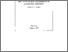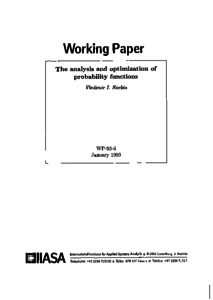# The Analysis and Optimization of Probability Functions

Norkin, V.I. (1993). The Analysis and Optimization of Probability Functions. IIASA Working Paper. IIASA, Laxenburg, Austria: WP-93-006Preview Text WP-93-006.pdf Download (927kB) | Preview

## Abstract

A problem of probability function optimization is considered. This function represents probability that some random quantity depending on deterministic parameters does not exceed some given level. The problem is motivated by studies of safety domains and risk control problems in complex stochastic systems. For example, pollution control includes maximization of probability that some given levels of deposition at reception points are not exceeded. Optimization of probability function is performed over a given range of parameters. To solve the problem stochastic quasi-gradient method is applied under quasi-concavity assumption on functions and measures involved. Convergence and rate of convergence results are presented.

Item Type: Monograph (IIASA Working Paper) Optimization under Uncertainty (OPT) IIASA Import 15 Jan 2016 02:02 27 Aug 2021 17:14 https://pure.iiasa.ac.at/3805View Item